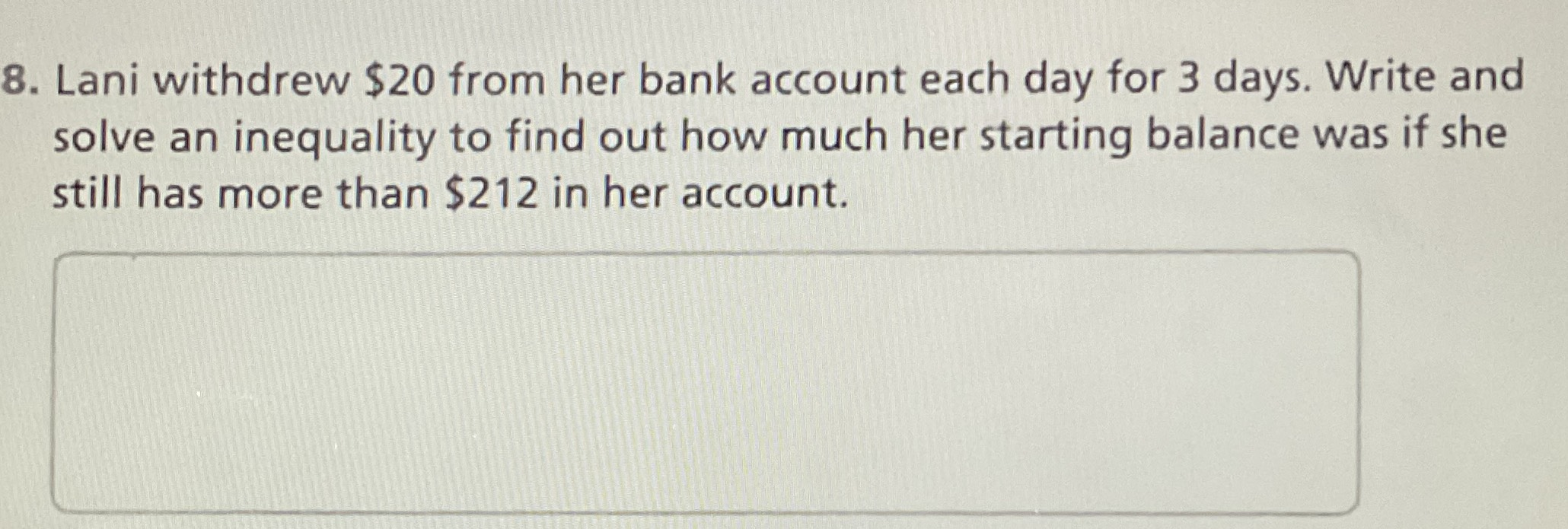### Still have math questions?

Algebra
Question8. Lani withdrew $$\ 20$$ from her bank account each day for $$3$$ days. Write and solve an inequality to find out how much her starting balance was if she still has more than $$\ 212$$ in her account.

$$x> 272$$